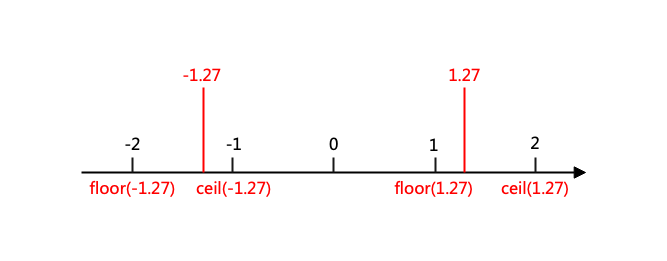# Python技法：浮点数取整、格式化和NaN处理

## 1.1 强转int类型

``````print(int(2.7)) # 2
print(int(-2.7)) # -2
``````

## 1.2 采用math.ceil和math.floor``````print(math.ceil(-1.27)) # -1
print(math.floor(-1.27)) # -2
print(math.ceil(1.27)) # 2
print(math.floor(1.27)) # 1
``````

## 1.3 采用round

round原型为`round(value, ndigits)`，可以将一个浮点数取整到固定的小数位。该函数对正数和负数都采取就近取整原则，而当某个值恰好等于两个整数间一半时，取整操作会取到离该值最近的那个偶数。像1.5和2.5这样的值都会取整到2。示例如下：

``````print(round(1.23, 0)) # 1.0
print(round(1.23, 1)) # 1.2
print(round(1.27, 1)) # 1.3
print(round(-1.27, 1)) # -1.3
print(round(1.25361, 3)) # 1.254
print(round(1.5, 0)) # 2.0
print(round(2.5, 0)) # 2.0
``````

``````a = 1627731
print(round(a, -1)) # 1627730
print(round(a, -2)) # 1627700
print(round(a, -3)) # 1628000
``````

## 2. 格式化浮点数输出

``````x = 1234.56789
s = format(x, "0.2f")
print(type(s), format(x, "0.2f")) # <class 'str'> 1234.57
``````

``````# 往右调整以对齐到10个字符
print(format(x, ">10.1f")) #     1234.6
# 往右调整以对齐到10个字符
print(format(x, "<10.1f")) # 1234.6
# 居中以对齐到10个字符
print(format(x, "^10.1f")) #   1234.6
# 增加千位分隔符
print(format(x, ",")) # 1,234.56789
# 增加千位分隔符并保存到1位小数
print(format(x, "0,.1f")) # 1,234.6

``````

``````print(format(x, "e")) # 1.234568e+03

print(format(x, "0.2E")) # 1.23E+03

``````

``````swap_separators = {ord("."):",", ord(","):"."}
print(format(x, ",").translate(swap_separators)) # 1.234,56789
``````

``````print("value is {:0.3f}".format(x)) # value is 1.235
print("The value is {:0,.2f}".format(x)) # The value is 1,234.57
``````

``````print("%.2f" % x)

print("%10.1f" % x)

print("%-10.1f" % x)
``````

## 3. 执行精确的小数计算

``````a = 2.1
b = 4.2
c = a + b
print(c) # 6.300000000000001
print(c==6.3) # False
print(round(c, 2)) # 6.3 企图这样修正精度（？？？）
``````

``````from decimal import Decimal

a = Decimal('4.2')
b = Decimal('2.1')
print(type(a + b), a + b) # <class 'decimal.Decimal'> 6.3
print((a + b) == Decimal('6.3')) # True
``````

``````print(type(a + 1), a + 1) # <class 'decimal.Decimal'> 5.2
``````

``````print((a + b) == 6.3) # False
``````

`decimal`模块的强大之处在于在计算过程中灵活地控制数字的位数和四舍五入，如我们可以创建一个本地的上下文环境然后修改精度的设定，如：

``````from decimal import localcontext
a = Decimal("1.3")
b = Decimal("1.7")
print(a/b) # 0.7647058823529411764705882353
with localcontext() as ctx:
ctx.prec = 3
print(a/b) # 0.765

with localcontext() as ctx:
ctx.prec = 50
print(a/b) # 0.764705882352941176470588235294117647058823529
``````

``````nums = [1.23e+18, 1, -1.23e+18]
print(sum(nums)) # 0.0
``````

``````import math
print(math.fsum(nums)) # 1.0
``````

## 4. 无穷大、负无穷大和NaN的判断测试

``````a = float("inf")
b = float("-inf")
c = float("nan")
print(a, b, c) # inf -inf nan
``````

``````print(math.isinf(a)) # True
print(math.isnan(c)) # True
``````

``````a = float("inf")
print(a + 45) # inf
print(a * 10) # inf
print(10/a) # 0.0
``````

``````a = float("inf")
print(a/a) # nan
b = float("-inf")
print(a + b) # nan
``````

NaN会通过所有的操作进行传播，且不会引发任何异常，如：

``````c = float("nan")
print(c + 23) # nan
print(c / 2) # nan
print(c + 2) # nan
``````

``````c = float("nan")
d = float("nan")
print(c == d) # False
print(c is d) # False
``````